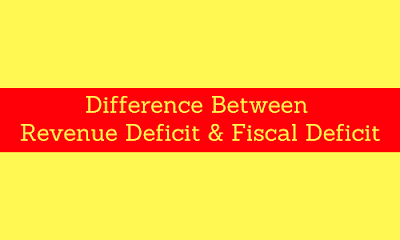# Difference Between Revenue Deficit & Fiscal Deficit### Introduction

Generally, the words are most commonly used in the budget, as you know budget itself means an estimation of income and expenditure well in advance.
One can manage their work whether it financial or not, the budget gives you an estimate from where the money comes from and where it goes.
And as you a budget is an estimation so it may never be the perfect so sometimes there may be surplus or deficit in it.
Deficit means an excess of expenditure over revenue in some short of time.
Revenue deficit
• A Revenue deficit is a difference between the revenue expenditure and the revenue receipts of the government treasury in a financial theory.
• It means, there is an excess of expenditure of revenue over the revenue receipts in a fiscal year.
• A Revenue deficit occurs when there is an imbalance between the revenue income and Expenditure.
• A revenue budget is estimated on the basis of previous year’s income and expenditure.
• So, Revenue deficit is a situation where the revenue falls and the expenses incurred as generally by nature.
• So, we can say that Revenue deficit is one kind of mismatch between the revenue and estimated expenditure.
• In simple language revenue deficit means the revenue doesn't match with the expenditure.

Revenue deficit = Revenue expenditure - revenue receipts.

### Fiscal deficit

• When the expenses for the period are higher than the actual revenue, it is known as the fiscal deficit.
• The fiscal deficit is a little bit same like revenue deficit but it covers the whole portion.
• Fiscal deficit includes revenue deficit along with those items which are excluded while calculating revenue deficit.
• It is defined as the imbalance between total expenditure over total receipts excluding last year’s borrowings.
• There are some reasons why fiscal deficit occurs are like an unexpected expenditure, natural disaster etc.

Fiscal deficit = Total Expenditure - total receipts (excluding borrowings)

Basis
Revenue deficit
Fiscal deficit
Meaning
It is the excess of total expenditure over total receipts.
It is the excess of revenue over the receipts.
Measures
Revenue deficit measures the inability of the government to meet its regular needs.
It measures the total borrowings needed by the government.
How to control
The government can control the revenue deficit by increases the tax and non- tax resources.
In this situation, the government may borrow the money or to print new currency.
Formula
Revenue deficit = Revenue expenditure - revenue receipts.
Fiscal deficit = Total Expenditure - total receipts (excluding borrowings)
Example
If in a budget you thought that income may be of Rs 100000, but at the end of a financial year you just earn Rs 75000. So the gap between projected earnings and actual earning is called revenue deficit which is Rs 25000 in this case.
If in a budget you thought that an expenditure may be of Rs 100000, but you thought that the income may be of Rs 75000. So the gap between projected expenditure and expected earning is called fiscal deficit which is Rs 25000 in this case.#### What's trending in BankExamsToday

Smart Prep Kit for Banking Exams by Ramandeep Singh - Download here